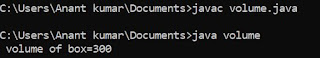## Friday, 23 November 2018

### Program in C++ to print digit pattern according to input number from user

(1.)  For printing this type structure in C++:

1
1 2
1 2 3
1 2 3 4
1 2 3 4 5

Here as we seen the figure is in row and column form thus we have to declare two variable ( i and j ) for row and column respectively. Here we use for loop statement of c++.

/* program to print structure */
#include<iostream.h>
#include<conio.h>
void main()
{
int i,j,n ;
clrscr () ;
cout<<"\n Enter number of rows:" ;
cin>>n;
for (i=1;i<=n;i++)
{
for (j=1;j<=i;j++)
{
cout<<"J":
}
cout<<"\n" ;
}
getch () ;
}

Output screen ::

Enter number of rows :: 5

output ::

1
1 2
1 2 3
1 2 3 4
1 2 3 4 5

(2.)   For printing this type structure in C++:

1
2 2
3 3 3
4 4 4 4
5 5 5 5 5

Here as we seen the figure is in row and column form thus we have to declare two variable ( i and j ) for row and column respectively. Here we use for loop statement of c++.

/* program to print structure */
#include<iostream.h>
#include<conio.h>
void main()
{
int i,j,n ;
clrscr () ;
cout<<"\n Enter number of rows:" ;
cin>>n;
for (i=1;i<=n;i++)
{
for (j=1;j<=i;j++)
{
cout<<"i";
}
cout<<"\n";
}
getch () ;
}

Output screen ::

Enter number of rows :: 5

output ::

1
2 2
3 3 3
4 4 4 4
5 5 5 5 5

(3.)  For printing this type structure in C++:

1
2 3
4 5 6
7 8 9 10
11 12 13 14 15

Here as we seen the figure is in row and column form thus we have to declare two variable ( i and j ) for row and column respectively. Here we use for loop statement of c++.

/* program to print structure */
#include<iostream.h>
#include<conio.h>
void main()
{
int i,j,n,number=0;
clrscr () ;
cout<<"\n Enter number of rows:" ;
cin>>n;
for (i=1;i<=n;i++)
{
for (j=1;j<=i;j++)
{
number++;
cout<<"number" ;
}
cout<<"\n" ;
}
getch () ;
}

Output screen ::

Enter number of rows :: 5

output :

1
2 3
4 5 6
7 8 9 10
11 12 13 14 15

## Featured post

### Program to find Volume of given box in java

class volume { public static void main (String args []) { int l=5,b=6,h=10; int volume; volume=l*b*h; System.out.println (" vol...